Home | Study Links | Poems |

## Primes and Patterns

Primes are the atoms and basic building blocks of mathematics. The challenge for hundreds of years has been to decide whether a given number is a prime or not, and to find a pattern in the distribution of the primes.
I shared my findings of definite and obvious patterns February 2014 at Yukon College (YRC-dept).Instead of looking for a pattern in the prime numbers I began looking for it in the non-prime numbers.When lining up all odd and even numbers in this manner (Chart A), I observed that the #3-frequency contained a repeating pattern occurring at regular intervals of 6.
Numbers colored blue and green are non-prime numbers and the prime numbers are displayed as orange.Clarified soon, frequency #1 represents both prime and non-prime numbers, and all even numbers (including 2) represent partitions separating the odd numbers. Imagine opposite sides of a zipper, odd and even numbers.The spacings between the twin prime sets such as 11/13 and 17/19, consist of multiples of 3.When lining up the sections horizontally, a zigzag pattern forming a frequency wave and consisting entirely of non-prime numbers became apparent, with numbers bouncing from 3 to 9 to 15- etc. at regular intervals of 6.
The appearance of this strip pattern brought to mind objects such as, a ribbon, tube, high-speed camera, movie filmstrip, and a railroad track. The vertical spacing's are added for easier viewing!The finding of #3-frequency, was quickly followed by the #5-frequency pattern.From here I continued with 20 or so additional sequential frequency waves as shown on the large display board.
This strip in (2-d) or tube (3-d) however long, represents the entire electromagnetic (EM) radiation spectrum. It consists of electromagnetic waves that oscillate both more rapidly and more sluggishly than visible light.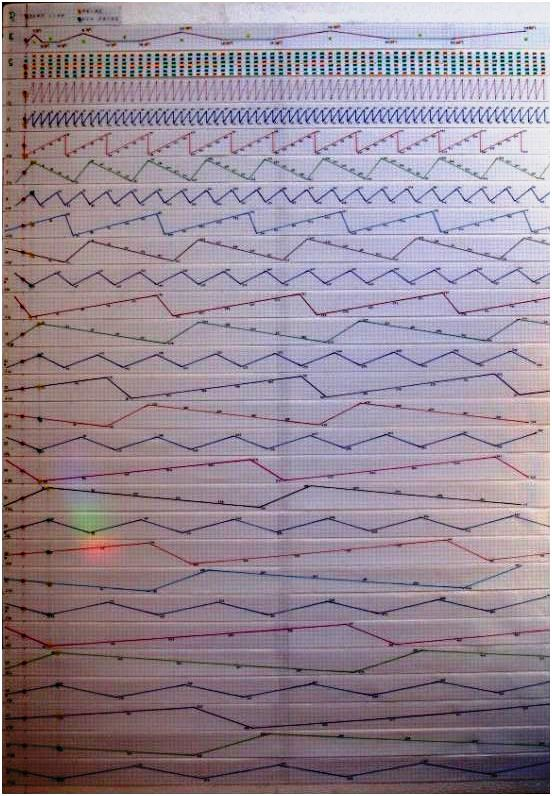If prime numbers are like the stars in the sky, non-prime numbers represent the space surrounding them. Consisting of electromagnetic spectrum frequency waves they are like finely woven threads of fabric and represent space and time. Australian aboriginals look for patterns in spaces between stars instead of patterns in the stars.

Another analogy is that prime numbers are like musical notes and non-prime numbers describe their vibrational wave patterns in a very exact and predictable manner. To know the note, you must first hear the sound!The #1- frequency wave includes all of the numbers on the strip, both prime and non-prime numbers. All other frequency waves consist of non-prime numbers only, except for the very first number beginning each prime frequency wave! Frequencies 3-5-7, the first few primes, cover most of the non-prime numbers on the entire strip.

The numbers belonging to the #3-frequency as mentioned earlier, occur at intervals of 6. The #5-frequency has intervals of 10, number 7 has 14, number 9 has 18, and so on. As you can see it increases by 4 each time.
For example 3-9-15 …, 5-15-25…, 7-21-35…, 9-27-45… etc.These frequencies consist of non-prime numbers overlaying the #1-frequency 'C' strip, thus sifting or separating out all of the primes. As the frequency numbers increase in size, meaning the very first number beginning each frequency wave after the zero point as represented by the red pins, their wave segments stretch accordingly. Furthermore, the frequencies and their segments or wave sections, match each other in specific ways. For example five #7-frequency segments = seven #5-frequency wave segments. Five #11-frequency segments = eleven #5-frequency wave segments. Seven #11-frequency segments = eleven #7-frequency wave segments. Etc.
The frequencies contain many interesting patterns.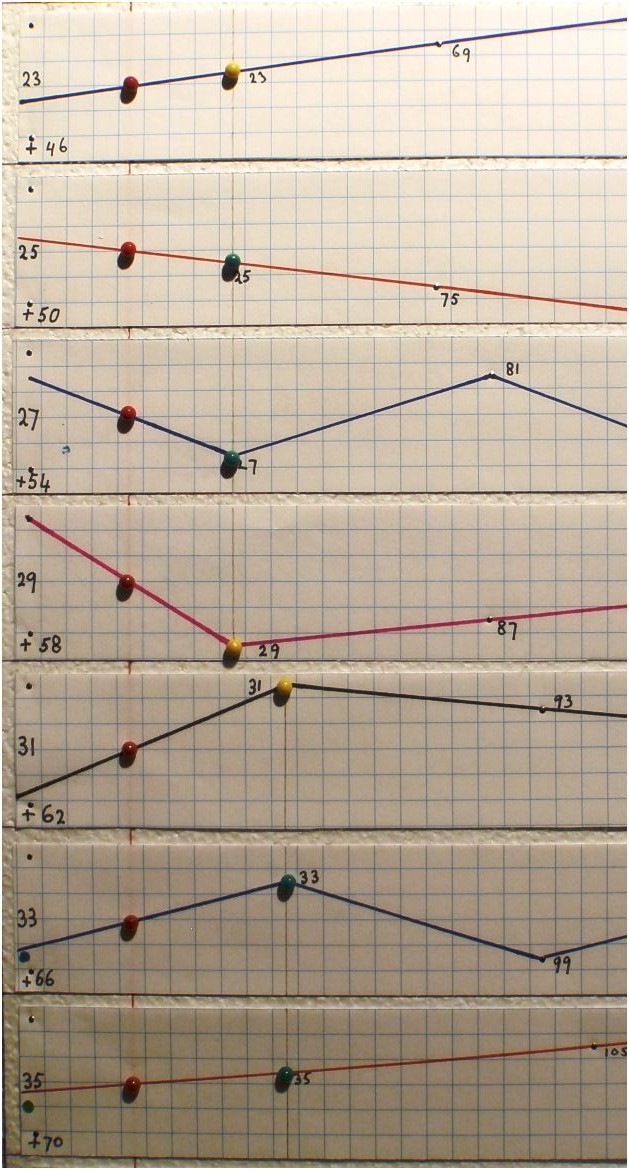By knowing the shape and arrangements of the frequency waves, one can predict prime numbers a million miles down the strip. Nevertheless, how to know exactly at which points along the strip new frequencies are required is explained with this important discovery: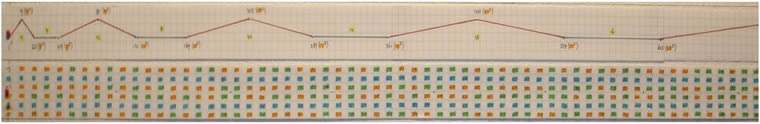The Pyramid strip, matches the 'C' strip. It is an essential tool for determining where exactly and how far along the 'C'- strip the various frequency insertion points are located, depending on the need to know the size of the numbers. The pyramids stretch horizontally with predictable increases, while their heights remain unchanged.Again, the #3-frequency covers all other related frequencies such as 9-15-21-27-etc, so that after the first small pyramid 1-9-25 no other pyramid peaks are required, and only the bottom line numbers are used from here on in.
The spacing ratio for the bottom line is 12-52-72-112-132-172-192-232- 252 etc., (4-2-4-2-4-2-etc).
Alternatively as ratios 2-1-2-1-2-1 or 1-0-1-0-1-0 etc. However, 252 and all numbers that are part of the prime 5 frequency sitting on these ‘ratio points’ could also be eliminated, but the consistent 4-2-4-2-4 pattern would be lost. This idea continues with the next frequency number 7 and 492 for example.The pyramid bases run along the strip at the same consistent level, 3 squares up and 4 down.Demonstration using the ''strip board'' (prime numbers are colored orange). I made transparent strips for the first few frequencies that will overlay each other on the #1-frequency strip board.Begin by placing the #3 frequency on the 1 board, which fills in all of the non-prime numbers to 25 (52).The #5 frequency fills in the non-prime numbers to number 49 (72), leaving only prime numbers marked as orange.Frequency #7 to 121 (112)Frequency #11 to 169 (132)Frequency #13 to 289 (172)Place frequency #17 and continue like this.

As you can see it effectively separates and sifts out the prime numbers from the non-prime numbers.
Example: At number 25 (52) enter frequency #5, at number 49 (72) enter frequency #7, and so on.

Imagine a train moving along a train track and passing known and predetermined stops along the way.
At each stop a new frequency is engaged to cover all non-prime numbers on the strip up to the next stop and ahead of the train. As the distances between stops increase continually the train can speed up since there are fewer stops and frequencies required. Again, distance and speed increases but the frequency numbers decrease. In other words, placing progressively longer sections of track ahead of a faster and faster moving train.

Compare to the infinity π line and a thought-provoking postulation by David.

While the speed of light (the entire electromagnetic spectrum as represented by Frequency 1) is fixed, data transfer at the speed of gravity or time happens much faster then the speed of light regardless of mass. Scientists have proven that quantum data/information is 10,000 times faster than the speed of light.
At nearly 3 trillion meters per second, i.e. instantaneous, because all data/information old or new, past and future already exists in the present, and prime numbers represent that data/information.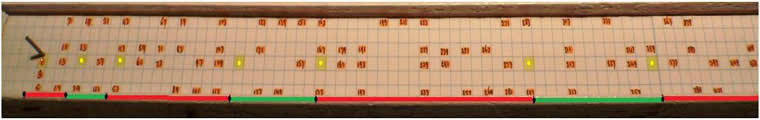The measurements for the stops all along the strip-board increase at predictable intervals. For example Red = 1”-3”-5”-7”-9”-etc, and green sections 1”-2”-3”-4”-5”-etc. (The paper strip has 4 squares per inch)What Riemann observed in his mathematics were the peaks and valleys of frequency waves with a common line running through them, the zero line. (Image taken from the The story of Math DVD)The Frequency waves are separated for display purposes, and the line of zeros appears to lie in a straight line.

Compare the vertical sections such as section 7 to 17 on the strip below, with the separated frequency layouts on the board. Observe how the frequency waves are shaped and how the 6 frequency line sections merge to points on the left side of the so-called zero line. Those points west of the zero line show-increasing intervals of 3”. E.g., frequency lines 11 and 13 merge at 3” west of the zero line and lines 23 and 25 merge 6” west of the zero line. Connecting those points on the left side of the zero line will produce a line intersecting with the zero line at -6, and tapering away from the zero line at 18.4°. The triangle it forms consists of angles 90°, 71.6°, and 18.4°.
Again, to this point the vertical spacing's were added for easier viewing!The #1-Frequency now correctly displayed without the vertical spacing's, forms a triangle of 90°, 80.5°, and 9.5°.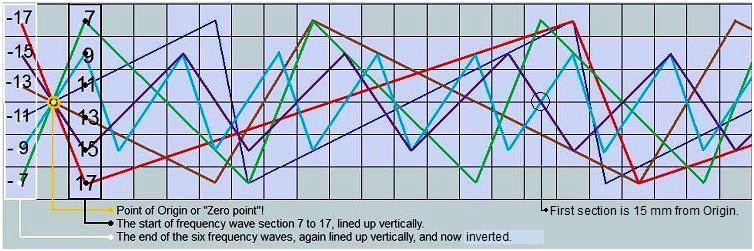You can see from the strip board pin (zero point), and this example of six frequencies (section 7 to 17), that all of the frequencies emanate from a single source point! Therefore, section 7 to 17 as the start of those 6 frequency waves, and section -17 to -7 mirroring those frequency waves, occur simultaneously.
This idea is described as a reversal point.

The simultaneous opposing positions between entangled quantum particles Photons, i.e. particles of light.

The distance the 6 frequency waves (7 to 17) are from the Origin before they line up vertically like they did at the start even though they are now inverted, is 382,882.5 mm x 2= 765,765 mm. (Squares are 1 mm)
(The LCM is 7 x 9 x 11 x 13 x 15 x 17= 2,297,295 / 6= 382,882.5)
1 section - 11 x 13= 143 mm------------x 5355= 765,765 (143 mm from point of Origin)
9 sections - 9 x 15= 135 mm--- 15 mm x 51051= 765,765 (15 mm from point of Origin)
1 section   - 7 x 17= 119 mm------------x 6435= 765,765 (119 mm from point of Origin)The distances increase like ripples in a circular pond for the subsequent wave sections such as 19-29, 31-41 etc.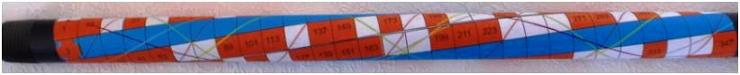This image presents the frequencies accurately as tubular, circular and spiralling, instead of flat and straight.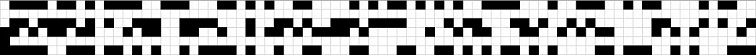Prime numbers, orange (3-d) and black (2-d), represent a melodic language.

This idea continues in DNA and Primes.

With this discovery and by knowing the frequency patterns, it is possible to decode encrypted numbers by finding the primes that created this number. Because without knowing the original primes it is almost impossible to decode that number. Simply locate the encoded number on the #1-frequency strip, and observe the prime frequencies attached to it. For example, the following numbers are the product of two prime numbers:

Product               Primes (frequency numbers)
15           =           3 x 5
77           =           7 x 11
221         =          13 x 17

Prime numbers, the Golden ratio spiral in 3-d plus time, where the 0 point represents the start and finish:Looking into the tube or frequencies toward the zero point, is like looking into the Golden ratio spiral with its excentric or concentric spiral motion, and comparable to the spiraling twist found inside rifle barrels.
The #1-frequency represents the entire golden ratio spiral, 1-3-5-7 etc., with each added frequency @ c forming increasingly complex geometric and fractal like repeating patterns.

The 3-frequency makes ¾ turns 3-9-15 etc. (Example section 9 to 15 in blue.)
The 5-frequency makes 1-1/4 turns, 5-15-25 etc.
The 7-frequency makes 1-3/4 turns, 7-21-35 etc.Connecting the numbers in 2-d belonging to each frequency as was explained earlier:
Prime #1= 1-3-5-7-9-etc. #3= 3-9-15-21-27-etc. #5= 5-10-15-25-35-etc. #7= 7-21-35-49-63-etc. etc.
Taking into account the two-opposite spiral motions and 3-d view, it would be like looking into a kaleidoscope.

Several ideas and questions that came to mind while working on this material:

• Twin prime frequencies such as 11 and 13 have a relationship because they exist next to each other. Observe the mirror image qualities (shown earlier) of twin prime frequency patterns as they emanate from, or intersect the zero point, like a mirror's reflection.
• My earlier work describes Planet diameters, ratios, and the Pythagorean drawing, where the Earth and the Moon have respective size ratios of 11 and 3. So I considered the following. If the earth has let's say an 11 frequency, then perhaps twin prime frequency-13 represents an earth twin like planet, which we could trace back to its source by following its own frequency wave. Therefore, instead of searching for planets randomly, it may be possible as in looking for a needle in a haystack, to follow the thread or frequency back to the needle which represents the planet.
• If we continue with the idea of the Earth and the Moon having respective frequencies of 11 and 3, then the point or source of these frequencies appears to be close by (represented by the pin), and it may just look as if Stars and Galaxies all around us move away from us in all directions! Perhaps the strip of numbers should be viewed as spherical or tube like with the zero point as its center.
• Imagine a tiny speaker from which originate all of the frequencies and information at the same time. Now take this idea a step further. Perhaps this information is not traveling from point A to B linear speaking, but our now experience of the micro and macrocosm is all contained within that single source point. Imagine a film not neatly wound up on a spool, but shaped as a ball, and to look upon like brain coral or brain exterior.
• Chart B shows twin primes, and the lines separating them suggests to me measured spectral rays and 'time lines' in the electromagnetic spectrum, which ranges from short gamma to long frequency radio waves. The spacing’s between them are multiples of 3 and can be measured accurately.
• Question: If the frequencies are radio or sound waves, what do they sound like?
• Question: Cicadas have 17-year cycles. Do they chirp using the 17-frequency?
• The number 2 is considered a prime number. However, I now view odd and even numbers as the two sides of a zipper. Perhaps the role of even numbers is to indicate increments like the clicks of a dial on a microscope for example, focusing in and out. Odd numbers may denote the unseen fabric of everything as represented by the complete range of frequency waves, and prime numbers the view or things visible such as stars and planets.Chart G shows another method to separate prime numbers from non-prime numbers. All multiplied numbers that appear in the center are non-prime numbers. Observe as well the bunching up and pattern forming of non-prime numbers in the upper corner of the sheet of paper. The sheet of paper (space) expands rapidly while the cluster of non-prime numbers in the corner gets smaller in relation, or giving the appearance of shrinkage.This next sieve method for finding prime numbers begins with several observations:

• The top row of numbers spaced at regular intervals of 6, (3 - 9 - 15 - etc.) represents the #3-frequency wave's non-prime numbers as identified in my first sieve find.
• Imagine the O in the top left corner as the point of Origin or zero point, and as the source of light.
• The 1 small square represents the main square (grid paper) however large it may be. So when looking towards the point of Origin, or the top left corner of the small square, it appears that this idea repeats itself going in both directions and who knows how often. With this information, try imagining the numbers as representing stars, the grid paper space or the universe, and the idea of identical and perhaps finite universes.
• Keep imagining that the blue lines are light rays passing through the grid paper, which has pinholes at the center of certain squares for the light to pass through. To repeat, the horizontal top row of numbers 3-9-15-21-etc. through which the blue lines pass through first, are numbers belonging to the #3-frequency wave.
• None of the squares representing the orange prime numbers, is ever intersected dead center by any blue frequency waves, only the non-prime number squares are, and so the prime numbers are free to drop out as in a Tetris game, down to the 45°- #3 diagonal line.
• The angle between the #3 and #9 lines is 26.565°. It is also the passageway angle within the Great Pyramid.• A new idea to ponder!• An exact angle of 21.9911...° (Π x 7), yields perfect ratios in the prime sieve by creating a constant ratio triangle, where section length A divided by B = 6.3, C divided by D = 2.1, and 6.3 divided by 2.1 = 3.
• This pattern and information is identical to the Stonehenge, Hunnebedden and Great Pyramid π pointer lines.
• An interesting question is how far out the blue π line will hit a horizontal/vertical grid point dead-on, if at all?The top corners for each square, representing frequency numbers 9, 15, 21, 27, etc. are evenly spaced as indicated by the black dots, and as you can see in the diagram all the other squares have similar arrangements.• All expanding right-angled triangles originate from the zero point in the upper left corner. Shown here are the first few right-angled triangles beginning the primes sieve. All triangles consist of non-prime numbers only.
• The first red right triangle 3² + 1.5²= 11.25 (A² + B²= C²), and so on.
• The small orange triangle 1² + .5² = 1.25 is distinct from the others, because it resides above all of the numbers! Again, the 1 small square in the upper left corner represents the entire main square (grid paper), or universe however large it may be, and it also points to the idea of identical and finite universes.• With this basic information showing an indefinite and regular progression of eight, the successive hypotenuses can be determined without much effort for however long one wants to work on this.Here I counted grid squares to show yet another regular and consistent pattern.To summarize all of the above information: The first nine squares represent all of the prime and non-prime numbers. The diagonal lines represent the frequencies as they cancel out all of the yellow non-prime numbers, leaving only the red prime numbers. Therefore, by knowing exact and predictable frequency patterns, mathematicians should be able to find a formula to see whether a given number is a prime or not.

The material in this web-page may help to clarify other outstanding areas concerning prime numbers such as the “Twin prime problem” and the “Goldbach conjecture”.

The amazing design and geometric precision in the distribution of the primes is such a Work of genius, that it should convince us that a supernatural intelligent Creator created this, and not a chaotic and ongoing “Big Bang explosion” out of which came perfect designs!